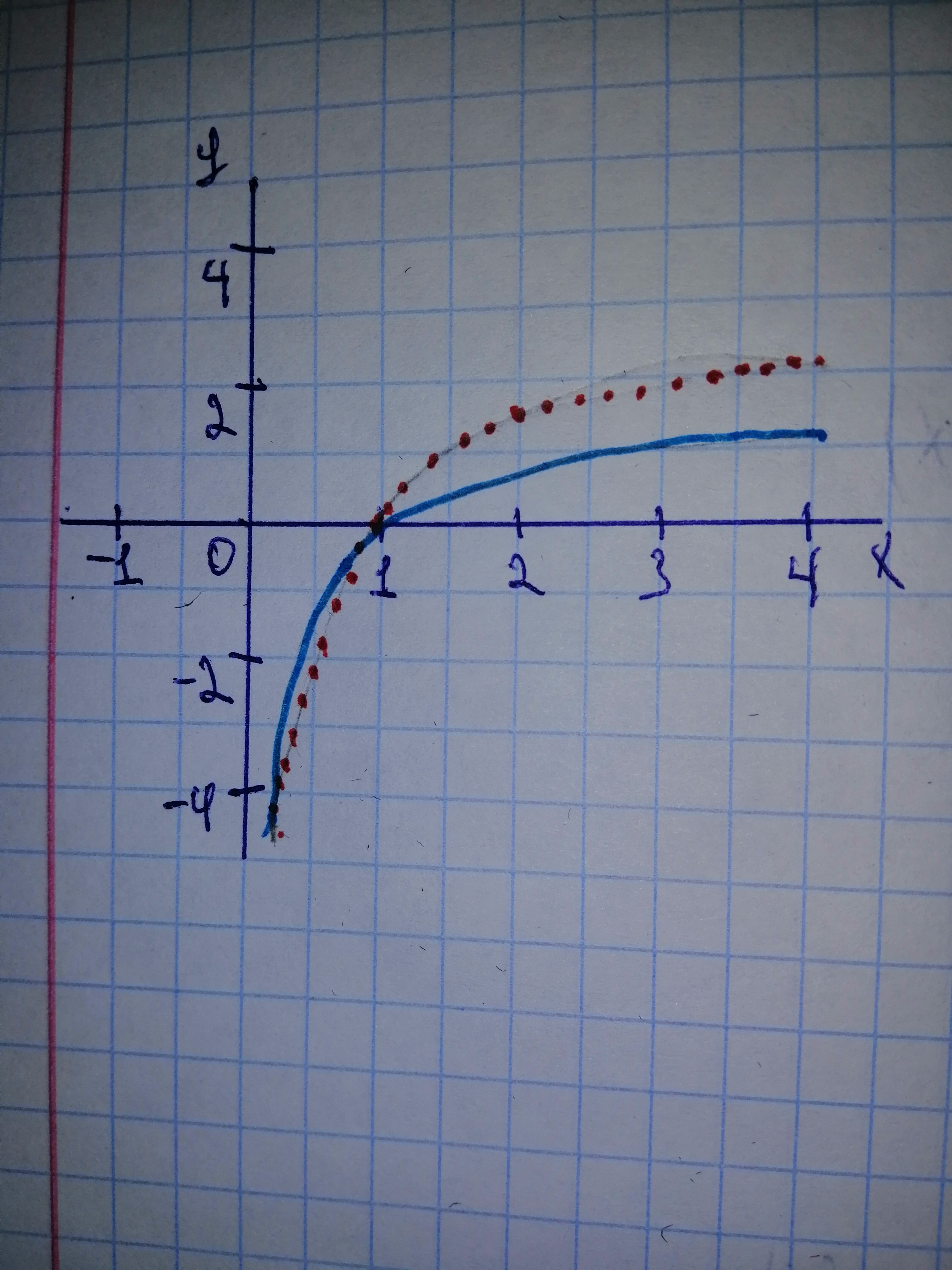# Begin by graphing f(x)= log_{2}x Then use transformations of this graph to graph the given function. What is the vertical asymptote? Use the graphs to determine each function's domain and range. g(x)=frac{1}{2} log_{2}xQuestion
Transformations of functionsBegin by graphing $$\displaystyle{f{{\left({x}\right)}}}=\ {{\log}_{{{2}}}{x}}$$ Then use transformations of this graph to graph the given function. What is the vertical asymptote? Use the graphs to determine each function's domain and range. $$\displaystyle{g{{\left({x}\right)}}}={\frac{{{1}}}{{{2}}}}\ {{\log}_{{{2}}}{x}}$$2020-11-13
Step 1Step 2 The dotted curve is the graph of $$\displaystyle{{\log}_{{{2}}}{x}}$$. The graph of $$\displaystyle{\frac{{{1}}}{{{2}}}}{{\log}_{{{2}}}{\left({x}\right)}}\ \text{is the graph of}\ {{\log}_{{{2}}}{x}}\ \text{stretched vertically by a scale factor of}\ {\frac{{{1}}}{{{2}}}}.$$ Its asympote is the line $$\displaystyle{x}={0}$$. It can be seen from the graph that the domain of the function is the set of all x to the right of the asymptote, that is $$\displaystyle{x}\ {>}\ {0}$$. The range of the function is the whole of $$\displaystyle{\mathbb{{{R}}}}$$.

### Relevant QuestionsBegin by graphing
$$\displaystyle{f{{\left({x}\right)}}}={{\log}_{{{2}}}{x}}$$
Then use transformations of this graph to graph the given function. What is the vertical asymptote? Use the graphs to determine each function s domain and range.
$$\displaystyle{g{{\left({x}\right)}}}={\frac{{{1}}}{{{2}}}}{{\log}_{{{2}}}{x}}$$Begin by graphing $$\displaystyle{f{{\left({x}\right)}}}=\ {{\log}_{{{2}}}{x}}$$ Then use transformations of this graph to graph the given function. What is the vertical asymptote? Use the graphs to determine each function's domain and range. $$\displaystyle{g{{\left({x}\right)}}}=\ -{2}\ {{\log}_{{{2}}}{x}}$$Begin by graphing $$\displaystyle{f{{\left({x}\right)}}}={{\log}_{{{2}}}{x}}$$ Then use transformations of this graph to graph the given function. What is the vertical asymptote? Use the graphs to determine each functions domain and range. $$\displaystyle{g{{\left({x}\right)}}}=\ -{2}{{\log}_{{{2}}}{x}}$$Begin by graphing $$\displaystyle{f{{\left({x}\right)}}}=\ {{\log}_{{{2}}}{x}}$$ Then use transformations of this graph to graph the given function. What is the graph's x-intercept? What is the vertical asymptote? Use the graphs to determine each function's domain and range. $$\displaystyle{g{{\left({x}\right)}}}=\ {{\log}_{{{2}}}{\left({x}\ -\ {2}\right)}}$$Begin by graphing $$\displaystyle{f{{\left({x}\right)}}}={{\log}_{{{2}}}{x}}$$ Then use transformations of this graph to graph the given function. What is the graph's x-intercept? What is the vertical asymptote? Use the graphs to determine each function's domain and range. $$\displaystyle{r}{\left({x}\right)}={{\log}_{{{2}}}{\left(-{x}\right)}}$$Begin by graphing
$$\displaystyle{f{{\left({x}\right)}}}={2}^{{{x}}}.$$
Then use transformations of this graph to graph the given function. Be sure to graph and give equations of the asymptotes. Use the graphs to determine each function's domain and range. If applicable, use a graphing utility to confirm your hand-drawn graphs.
$$\displaystyle{g{{\left({x}\right)}}}={\frac{{{1}}}{{{2}}}}\ \cdot\ {2}^{{{x}}}$$Begin by graphing
$$\displaystyle{f{{\left({x}\right)}}}={2}^{{{x}}}.$$
Then use transformations of this graph to graph the given function. Be sure to graph and give equations of the asymptotes. Use the graphs to determine each function's domain and range. If applicable, use a graphing utility to confirm your hand-drawn graphs.
$$\displaystyle{g{{\left({x}\right)}}}={2}^{{{x}\ +\ {2}}}$$Begin by graphing
$$\displaystyle{f{{\left({x}\right)}}}={2}^{{{x}}}.$$
Then use transformations of this graph to graph the given function. Be sure to graph and give equations of the asymptotes. Use the graphs to determine each function's domain and range. If applicable, use a graphing utility to confirm your hand-drawn graphs.
$$\displaystyle{g{{\left({x}\right)}}}={2}^{{{x}}}={2}^{{{x}}}\ +\ {2}$$$$\displaystyle{f{{\left({x}\right)}}}={2}^{{{x}}}$$
$$\displaystyle{g{{\left({x}\right)}}}=-{2}^{{{x}}}$$$$\displaystyle{f{{\left({x}\right)}}}={2}^{{{2}}}$$
$$\displaystyle{g{{\left({x}\right)}}}={2}^{{-{x}}}$$